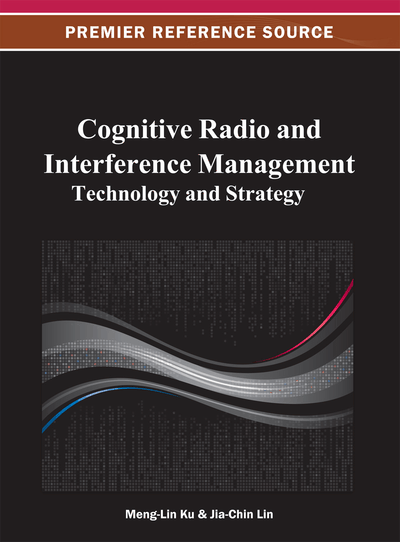# Robust Performance of Spectrum Sensing in Cognitive Radio Networks

Shimin Gong (Nanyang Technological University, Singapore), Ping Wang (Nanyang Technological University, Singapore) and Jianwei Huang (The Chinese University of Hong Kong, HKSAR, China)
DOI: 10.4018/978-1-4666-2005-6.ch003

## Abstract

Harmonic coexistence of cognitive radio systems and licensed systems requires the secondary users to have the capability of sensing and keeping track of primary users’ transmissions. While existing spectrum sensing methods usually assume known distributions of the primary signals, such an assumption is often not true in practice. As a result, applying existing sensing methods will often lead to unreliable detection performance in practical networks. In this chapter, the authors try to investigate the sensing performance under the distribution uncertainty of primary signals. They first investigate the performance bounds for single user detection with unknown distribution, and provide an analytical expression for the lower bound of detection probability. Moreover, they bring the distribution uncertainty into multi-user cooperative sensing. The authors formulate the optimal sensing design as a robust optimization problem, and propose an iterative algorithm to determine the optimal decision threshold for each user. Extensive simulations demonstrate the effectiveness of the proposed algorithm.
Chapter Preview
Top

## 3.1 Introduction

Traditionally, wireless spectrum has been statically allocated to license holders for exclusive use, and the spectrum available for new applications has become increasingly scarce and pricey. On the other hand, field measurement report (Mchenry, 2003) has revealed that the actual utilization is often quite low, e.g., some radio bands for TV broadcasting and industrial, scientific and medical (ISM) radio bands are idle most of the time. Under this situation, Cognitive Radio (CR) (Haykin, 2005) is proposed as a promising technique to improve spectrum utilization. In a CR system, secondary users (SUs) are allowed to access the licensed spectrum bands when they are temporarily unoccupied by primary users (PUs). A key technology to achieve this is spectrum sensing, where SUs need to determine the presence of PUs based on the characteristics of the measured signals on the channels.

There have been extensive studies on the design and performance analysis of spectrum sensing mechanisms. Generally, spectrum sensing is formulated as a hypothesis testing problem, which takes measured signal samples (possibly embedded with primary signals) as inputs and makes a decision by comparing the samples with a pre-designed decision threshold. The detection performance is usually measured by the receiver operating characteristic (ROC), which denotes the tradeoff between detection probability (i.e., probability of sensing a busy channel as busy) and false alarm probability (i.e., probability of sensing an idle channel as busy). Existing results usually assume that the received primary signal follows a Gaussian distribution, and express the detection probability in the-function (tail probability of the standard normal distribution) (Sousa, 2005) (Simon, 2007). Some other sensing schemes for multiuser cooperative sensing are analyzed through deterministic optimization problem (Sayed, 2008) (Taricco, 2011). For an overview study regarding the sensing techniques in cognitive radio networks, please refer to (Anant Sahai, 2004), (Sousa, 2005), (Li, 2008) and (Zhi Quan, 2008).

However, most of the existing studies assume that the statistical features (such as probability distribution function) of the primary signals received at SUs' receivers are precisely known beforehand, e.g., following Rayleigh, or Rician distributions. In fact, precise knowledge regarding the signal distribution is usually a very strong assumption, and does not necessarily lead to reliable decisions due to unpredictable factors under practical circumstance. On one hand, it is often hard to model a realistic distribution of the received signal in closed-form without significant simplifications. Even if a precise characterization is possible, a complex form usually makes the analysis computational demanding. On the other hand, a deterministic assumption may not always match the real distribution when spectrum environment fluctuates. For example, the received signals exhibit different distributions depending on whether there is line-of-sight between transmitter and receiver. Besides, uncertainty may also occur in the channel estimation. It further leads to unreliable distribution parameters and detection errors.

In this chapter, we will study robust spectrum sensing under distribution uncertainty of the primary signals. Specifically, we consider the case where the statistical features (i.e., mean and variance) of the primary signals are contaminated by estimation errors, and the actual probability density function (pdf) does not match what is often assumed in literature. To capture the distribution uncertainty, we define an uncertainty set that contains all possible signal distributions whose mean and variance are close to a nominal one, and then formulate the sensing design as a robust optimization problem. Solving the robust optimization problem in general is more difficult than solving its non-robust counterpart (also called nominal problem). Some researchers (Aharon Ben-Tal, 1998) (Aharon Ben-tal, 1998) (Teo, 2007) have studied the solvability of robust optimization and proposed some useful results that can be applied into practical problems.

## Complete Chapter List

Search this Book:
Reset